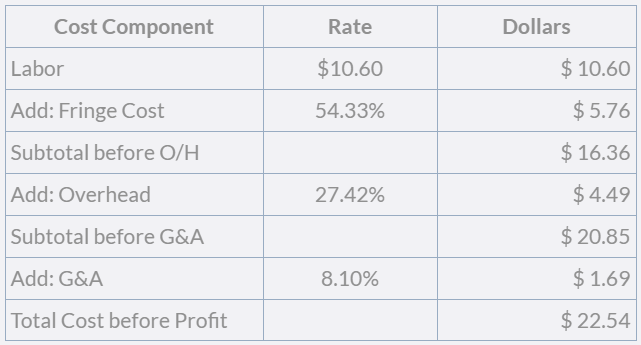# What is a Wrap Rate?For many companies who have the federal government as a customer, a key business statistic is the “wrap rate”.

A wrap rate is normally attributed to the pricing of the labor component of a contractor’s revenue model. The wrap rate provides a shortcut method of determining the cost of a unit of labor before profit is applied to yield a “sale price”. The wrap rate can be used as a quick method to determine the price competitiveness of the contractor and is particularly important to determine whether or not to respond to a Request for Proposal (RFP).

## How to Calculate a Wrap Rate

A “wrap rate” is a number. There are four calculations used to derive the wrap rate number:
1. A unit of labor (normally an hour) is multiplied by an employee’s base pay rate to derive a base dollar cost (for a unit of labor).

2. Indirect expense pools and allocation bases are applicable to the base labor cost you are bidding. The indirect expense pools and their allocation bases are calculation components of an indirect expense rate. Typically for labor, there is an application of three indirect expense rates: fringe, overhead, and G&A.

3. Next, using this “cost build-up” methodology to derive the wrap rate, that unit hourly base cost is multiplied by each of the applicable indirect expense rates to calculate allocated dollars to be added to the base labor rate.

4. By adding the indirect cost dollars to the base dollar cost, you come up with a total cost of the hourly labor unit. This number is the numerator component of a wrap rate. The denominator is the per-unit cost of labor.

## A Detailed Example

Suppose you are bidding on a federal contract that is covered under Service Contract Labor Standards or by its more widely used name, the Service Contract Act (SCA). This means the Department of Labor has predetermined the "health and welfare" or fringe benefits to be paid to each non-exempt employee who works on the contract. The federal contractor minimum wage is currently \$10.60 per hour, and we will assume that will be the starting rate of pay for new hires on this SCA covered contract.

1. Federal Contractor Minimum Wage = \$10.60 per hour
2. Add Employer FICA and Medicare = \$0.81 per hour
3. Add Health and Welfare = \$4.54* per hour
4. Add Paid Leave = \$0.41 per hour
5. Determine the Indirect Expense Rate

1. Fringe Pool (unitized) = .81+4.54+.41 = \$5.76
2. Fringe Base (unitized) = \$10.60
3. Fringe Rate = a/b
4. Fringe Rate = 54.33%

## Apply Pre-determined Overhead and G&A Rates

Now that you have calculated a rate with only fringes applied to the base pay rate, it would be most beneficial to utilize an accounting software package such as SYMPAQ to calculate your year-to-date actual indirect rates for your overhead and G&A expenses. This is essential for calculating the wrap rate using the “cost build up” method. After calculating your indirect rates for overhead and G&A - for illustrative purposes – let’s say those calculations result in a government site overhead burden of 27.42% and a G&A burden of 8.10%, respectively.

The table below provides an illustration of the cost build up method:

1. Build your indirect cost pools and allocation bases to determine an indirect expense rate associated with each indirect cost layer. In this example, the ‘building’ of the pools and bases is not discussed. Each indirect cost layer will have a rate calculated that is expressed as a percentage.2. Calculate the wrap rate by dividing the direct and indirect expense rates into the labor to derive one “multiplier”.

Wrap Rate = Total Cost (\$22.54) /Base Cost of Labor (\$10.60) = 2.1263

From this example, you can see that an employee who is getting paid the minimum government contract wage of \$10.60 per hour is yielding a wrap rate that is 2.1263 times the hourly rate of pay.

* Effective July 5, 2019, if a contractor provides a minimum of 56 hours of sick time, then the H&W rate is \$4.22/hr. If not, the H&W rate is \$4.54/hr.